# NB-IoT NTN NPDSCH Throughput

This example shows how to perform a narrowband Internet of Things (NB-IoT) narrowband physical downlink shared channel (NPDSCH) throughput simulation in a non-terrestrial network (NTN) channel. This example supports these two narrowband NTN channels.

• European Telecommunication Standards Institute (ETSI) Rician fading channel

• International Telecommunication Union Radiocommunication Sector (ITU-R) P.681 land mobile-satellite (LMS) channel

### Introduction

This example measures the NPDSCH throughput of an NB-IoT link, as defined by the 3GPP NB-IoT standards , , , , and .

The example models these features.

• Transport channel coding

• NPDSCH, narrowband reference signal (NRS), and synchronization signals (narrowband primary synchronization signal NPSS, narrowband secondary synchronization signal NSSS)

• ETSI Rician channel and ITU-R P.681 LMS channel

• Doppler pre-compensation at the transmitter, and Doppler compensation at the receiver

• Optional power amplifier modeling

The figure shows the implemented processing chain. For clarity, the figure does not show the NRS and synchronization signals.For more information about the NB-IoT NPDSCH transmitter and receiver processing units, see the NB-IoT NPDSCH Block Error Rate Simulation (LTE Toolbox) example.

To reduce the total simulation time, you can use Parallel Computing Toolbox™ to execute the range of transmit power values of the transmit power loop in parallel.

### Configure Simulation Length, Transmitter, and Receiver

Set the length of the simulation in terms of the number of transport blocks. By default, the example uses 10 transport blocks, but you must use a large number of transport blocks to produce meaningful throughput results. Set the range of transmit power values to simulate. The transmitter power is defined as the power of the time-domain waveform before passing to the power amplifier. The receiver includes its noise figure and the antenna temperature. The noise figure models the receiver internal noise, and the antenna temperature models the input noise. This receiver specifies the noise per antenna element. In this example, you perform the simulation for different repetition values and compare the performance improvement with repetitions. The `iReps` variable is applicable only when NPDSCH does not carry the scheduling information block SIB1-NB.

```numTrBlks = 10; % Number of simulated transport blocks iReps = [0 5]; % Range of repetitions simulated txPower = 30:5:45; % Transmit power (dBm) rxNoiseFigure = 6; % Noise figure (dB) rxAntennaTemperature = 290; % Antenna temperature (K)```

### Power Amplifier Configuration

To configure a memoryless power amplifier nonlinearity, use `enablePA`. You can choose one of these power amplifier models, as defined in Annex A of TR 38.803.

• 2.1 GHz Gallium Arsenide (GaAs)

• 2.1 GHz Gallium Nitride (GaN)

Alternatively, you can set `paModel` to `Custom` and use `paCharacteristics` to define the power amplifier characteristics in a matrix with three columns. The first column defines the input power in dBm. The second column defines the output power in dBm. The third column defines the output phase in degrees. When the `paCharacteristics` variable is set to empty and the `paModel` is set to `Custom`, this example uses a 2.1 GHz laterally-diffused metal-oxide semiconductor (LDMOS) Doherty-based amplifier.

The memoryless nonlinearity applied to the waveform follows this equation for power amplifiers (excluding a custom configuration).

`${\mathit{y}}_{\mathit{P}}\left(\mathit{n}\right)=\sum _{\mathit{k}\text{\hspace{0.17em}}\in {\text{\hspace{0.17em}}\mathit{K}}_{\mathit{p}}}{\mathit{a}}_{\mathit{k}}\text{\hspace{0.17em}}\mathit{x}\left(\mathit{n}\right)\text{\hspace{0.17em}}{|\mathit{x}\left(\mathit{n}\right)|}^{2\mathit{k}}$`

In this equation,

• ${\mathit{y}}_{\mathit{P}}\left(\mathit{n}\right)$ is the output signal.

• $\mathit{x}\left(\mathit{n}\right)$ is the input signal.

• ${\mathit{K}}_{\mathit{p}}$ is the set of polynomial degree(s).

• ${\mathit{a}}_{\mathit{k}}$ is the polynomial coefficient.

By default, the example sets `enablePA` to `false`.

```enablePA =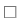false; % true or false paModel ="2.1GHz GaAs"; % "2.1GHz GaAs", "2.1GHz GaN", or "Custom" paCharacteristics = []; % Lookup table as empty or a matrix with columns: Pin (dBm) | Pout (dBm) | Phase (degrees) % If enablePA is set to true, visualize the power amplifier gain and phase % characteristics paModelImpl = paModel; if enablePA == 1 % Set the power amplifier as applicable for the further processing if lower(paModel) == "custom" if isempty(paCharacteristics) tableLookup = getDefaultCustomPA; else tableLookup = paCharacteristics; end % Use table look-up option of comm.MemorylessNonlinearity and provide % the power amplifier characteristics mnl = comm.MemorylessNonlinearity(Method="Lookup table", ... Table=tableLookup); plot(mnl) paModelImpl = mnl; else paMemorylessNonlinearity(paModel) end end```

### Doppler Compensation Configuration

The example supports two Doppler compensation configurations: one at the transmitter end and the other at the receiver end. For compensation at the transmitter end, enable `txDopplerCompensator`. Setting the `txDopplerCompensator` variable to `true` pre-compensates the transmitted waveform for Doppler caused by the satellite movement. For compensation at the receiver end, enable `rxDopplerCompensator`. Setting the `rxDopplerCompensator` variable to `true` performs Doppler compensation at the receiver using NPSS. Note that, in the case of Doppler compensation at the receiver, if the operating SNR is below -10 dB, the Doppler estimates can be inaccurate.

```txDopplerCompensator =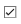true; % true or false rxDopplerCompensator =false; % true or false```

### Set Up Higher Layer Parameters

To configure the eNodeB and NPDSCH parameters, set these higher layer parameters.

```npdschDataType ="NotBCCH"; % "SIB1NB", "BCCHNotSIB1NB", or "NotBCCH" iSF = 0; % Resource assignment field in DCI (DCI format N1 or N2) schedulingInfoSIB1 = 0; % Scheduling information field in MasterInformationBlock-NB (MIB-NB) iMCS = 4; % Modulation and coding scheme field in DCI (DCI format N1 or N2)```

### eNodeB and NPDSCH Configuration

Set these eNodeB parameters.

• NB-IoT physical layer cell identity

• Operation mode

```enb = struct; enb.NNCellID = 0; % NB-IoT physical layer cell identity enb.OperationMode ="Standalone"; % "Standalone", "Guardband", "Inband-SamePCI", or "Inband-DifferentPCI"```

Set the radio network temporary identifier in the NPDSCH structure.

```npdsch = struct; npdsch.RNTI = 1; % Radio network temporary identifier```

### Propagation Channel Model Configuration

Create a channel model object for the simulation. The example supports the ETSI Rician channel and ITU-R P.681 LMS channel. To obtain the NTN channel from these channels, apply an additional Doppler shift. Use this equation to calculate the Doppler shift due to satellite movement, as specified in TR 38.811.

`${\mathit{f}}_{\mathit{d},\mathrm{sat}}=\left(\frac{{\nu }_{\mathrm{sat}}\text{\hspace{0.17em}}}{\mathit{c}}\right)*\left(\frac{\mathit{R}}{\mathit{R}+\mathit{h}}\text{\hspace{0.17em}}\mathrm{cos}\left({\alpha }_{\mathrm{model}}\right)\right)*{\mathit{f}}_{\mathit{c}}$`

In this equation,

• ${\nu }_{\mathrm{sat}}$ is the satellite speed.

• $\mathit{c}$ is the speed of light.

• $\mathit{R}$ is the Earth radius.

• $\mathit{h}$ is the satellite altitude.

• ${\alpha }_{\mathrm{model}}$ is the satellite elevation angle.

• ${\mathit{f}}_{\mathit{c}}$ is the carrier frequency.

By default, this example considers a satellite in low Earth orbit at an altitude of 600 km, with a carrier frequency of 2 GHz. The NB-IoT user equipment (UE) is moving at a speed of 3 km/h. Also, this example assumes that satellites move in circular orbits. Verify that the satellite speed and satellite altitude are in agreement with each other.

```channel = struct; channel.NTNChannelType =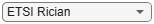"ETSI Rician"; % "ETSI Rician" or "ITU-R P.681" channel.CarrierFrequency = 2e9; % Carrier frequency (in Hz) channel.ElevationAngle = 50; % Elevation angle (in degrees) channel.MobileSpeed = 3*1000/3600; % UE speed (in m/s) channel.SatelliteSpeed = 7562.2; % Satellite speed (in m/s) channel.SatelliteAltitude = 600e3; % Satellite altitude (in m) channel.Seed = 73; % Random seed channel.IncludeFreeSpacePathLoss =true; % Include or exclude free space path loss % Set these fields based on the type of channel selected if lower(channel.NTNChannelType) == "etsi rician" % For ETSI Rician channel, set KFactor channel.KFactor = 10; % In dB else % For ITU-R P.681, set Environment and AzimuthOrientation channel.Environment = "Urban"; % "Urban", "Suburban", "RuralWooded", or "Residential" channel.AzimuthOrientation = 0; % In degrees end```

### Channel Estimator Configuration

Configure a practical channel estimator by using the `cec` structure. By default, this example configures the channel with these specifications.

• Carrier frequency — 2 GHz

• Speed of NB-IoT UE — 3 km/h

This configuration results in a Doppler spread of 5.5 Hz. Therefore, perform frequency averaging over pilot estimates with these settings.

• Time window — 1 resource element (RE)

• Frequency window — 25 REs, to ensure averaging over all subcarriers for the resource block

```% Configure channel estimator cec.PilotAverage = "UserDefined"; % Type of pilot symbol averaging cec.TimeWindow = 1; % Time window size in REs cec.FreqWindow = 25; % Frequency window size in REs cec.InterpType = "Cubic"; % 2-D interpolation type cec.InterpWindow = "Centered"; % Interpolation window type cec.InterpWinSize = 3; % Interpolation window size cec.Reference = "NRS"; % Channel estimator reference signal```

### Processing Loop

To determine the throughput at each repetition index and SNR index, follow these steps.

1. Generate the transport block — Get the transport block size depending on the configured higher layer parameters.

2. Generate the resource grid — Map the modulated bits, along with the NPSS, NSSS, and NRS, signals to the resource grid. The `lteNDLSCH` (LTE Toolbox) function performs transport channel coding on the input transport block. The `lteNPDSCH` (LTE Toolbox) function then modulates the encoded data bits.

3. Generate the waveform — Generate the NB-IoT time-domain OFDM waveform with half subcarrier shift using the `lteSCFDMAModulate` (LTE Toolbox) function.

4. Apply power amplifier nonlinearities — Apply the memoryless nonlinearities to the baseband OFDM signal.

5. Apply Doppler pre-compensation — Apply the Doppler shift due to satellite movement to the generated waveform to pre-compensate the channel-induced satellite Doppler shift.

6. Model and apply a noisy channel — Pass the generated waveform through an ETSI Rician or ITU-R P.681 LMS fading channel to get the faded waveform. Apply path loss and add thermal noise to the faded waveform.

7. Apply Doppler compensation — Estimate the Doppler shift in the received waveform, and compensate the Doppler shift.

8. Perform synchronization and OFDM demodulation — Perform timing synchronization by correlating the received waveform with the NPSS. The `lteSCFDMADemodulate` (LTE Toolbox) function then demodulates the synchronized signal.

9. Perform channel estimation — Estimate the channel using NRS.

10. Decode the NPDSCH — Decode the NPDSCH, with the estimated channel and noise variance, by using the `lteNPDSCHDecode` (LTE Toolbox) function.

11. Decode the transport block — Decode the soft bits using the `lteNDLSCHDecode` (LTE Toolbox) function. The function decodes the codeword data and returns the block cyclic redundancy check (CRC) error.

```% Use the higher layer parameters, and check if the provided configuration % is valid numRep = numel(iReps); npdschInfo = hNPDSCHInfo; npdschInfo.NPDSCHDataType = npdschDataType; npdschInfo.ISF = iSF; npdschDataTypeLower = lower(npdschDataType); if npdschDataTypeLower == "sib1nb" % NPDSCH carrying SIB1-NB npdschInfo.SchedulingInfoSIB1 = schedulingInfoSIB1; % Store a copy of the information structure for all the repetitions npdschInfo = repmat(npdschInfo,numRep,1); else % NPDSCH not carrying SIB1-NB npdschInfo.IMCS = iMCS; % Modulation and coding scheme field in DCI (DCI format N1 or N2) % Store a copy of the information structure for all the repetitions npdschInfo = repmat(npdschInfo,numRep,1); for repIdx = 1:numRep npdschInfo(repIdx).IRep = iReps(repIdx); % Repetition number field in DCI (DCI format N1 or N2) end end % Initialize some parameters of enb enb.NFrame = 0; enb.NSubframe = 0; enb.NBRefP = 1; opMode = lower(enb.OperationMode); inbandSamePCI = (opMode == "inband-samepci"); inbandDifferentPCI = (opMode == "inband-differentpci"); if inbandSamePCI enb.CellRefP = enb.NBRefP; % Number of cell RS antenna ports (Fixed to 1 in this example) enb.NCellID = enb.NNCellID; elseif inbandDifferentPCI enb.CellRefP = enb.NBRefP; % Number of cell RS antenna ports (Fixed to 1 in this example) enb.NCellID = 1; end if ((npdschDataTypeLower == "bccnnotsib1nb") || (npdschDataType == "notbcch")) && ... (inbandSamePCI || inbandDifferentPCI) enb.ControlRegionSize = 3; % The allowed values are 0...13 end % Apply default window size according to TS 36.104 Table E.5.1-1a if(~isfield(enb,"Windowing")) enb.Windowing = 6; end % Store enb structure with a name used for OFDM modulation and % demodulation. The NB-IoT downlink waveform is a 1/2 subcarrier shift % waveform. The lteSCFDMAModulate and lteSCFDMADemodulate functions use the % NBULSubcarrierSpacing field to modulate and demodulate the NB-IoT % downlink waveform, respectively. enbOFDM = enb; enbOFDM.NBULSubcarrierSpacing = "15kHz"; % Get the waveform information and set up the NTN channel waveformInfo = lteSCFDMAInfo(enbOFDM); ntnChannel = setupNTNChannel(channel,waveformInfo.SamplingRate); % Compute the noise amplitude per receive antenna kBoltz = physconst('Boltzmann'); NF = 10^(rxNoiseFigure/10); T0 = 290; % Noise temperature at the input (K) Teq = rxAntennaTemperature + T0*(NF-1); % K N0_ampl = sqrt(kBoltz*waveformInfo.SamplingRate*Teq/2.0); % Compute path loss based on the elevation angle and satellite altitude re = physconst("earthradius"); c = physconst("lightspeed"); h = channel.SatelliteAltitude; elevAngle = channel.ElevationAngle; d = -re*sind(elevAngle) + sqrt((re*sind(elevAngle)).^2 + h*h + 2*re*h); lambda = c/channel.CarrierFrequency; pathLoss = fspl(d,lambda)*double(channel.IncludeFreeSpacePathLoss); % in dB % Initialize throughput result numTxPow = numel(txPower); throughputPercent = zeros(numTxPow,numRep); % Absolute subframe number at the starting point of the simulation NSubframe = enb.NFrame*10+enb.NSubframe; % Loop over repetitions repVal = zeros(numRep,1); for repIdx = 1:numRep % Add these fields to the npdsch structure npdsch.NSF = npdschInfo(repIdx).NSF; npdsch.NRep = npdschInfo(repIdx).NRep; npdsch.NPDSCHDataType = npdschDataType; repVal(repIdx) = npdsch.NRep; % Get the bit capacity and transport block length [~,info] = lteNPDSCHIndices(enb,npdsch); rmoutlen = info.G; % Bit length after rate matching (codeword length) trblklen = npdschInfo(repIdx).TBS; % Transport block size % The temporary variables 'enb_init', 'enbOFDM_init', and % 'channel_init' create the temporary variables 'enb', 'enbOFDM', and % 'ntnChannel' within the SNR loop to create independent simulation % loops for the 'parfor' loop enb_init = enb; enbOFDM_init = enbOFDM; channel_init = ntnChannel; for txPowIdx = 1:numTxPow % parfor txPowIdx = 1:numTxPow % To enable the use of parallel computing for increased the speed, % comment out the 'for' statement and uncomment the 'parfor' statement. % This functionality requires the Parallel Computing Toolbox. If you do % not have Parallel Computing Toolbox, 'parfor' defaults to the normal % 'for' statement. % Reset the random number generator so that each transmit power % point experiences the same noise realization rng(0,"threefry"); enb = enb_init; % Initialize eNodeB configuration enbOFDM = enbOFDM_init; % Initialize eNodeB configuration related to OFDM waveform ntnChannel = channel_init; % Initialize fading channel configuration txcw = []; % Initialize the transmitted codeword numBlkErrors = 0; % Number of transport blocks with errors estate = []; % Initialize NPDSCH encoder state dstate = []; % Initialize NPDSCH decoder state lastOffset = 0; % Initialize overall frame timing offset offset = 0; % Initialize frame timing offset subframeGrid = lteNBResourceGrid(enb); % Initialize the subframe grid foffsetRS = 0; % Initialize frequency offset using reference signal N0 = N0_ampl; pl_dB = pathLoss; subframeIdx = NSubframe; numRxTrBlks = 0; reset(ntnChannel.BaseChannel); reset(ntnChannel.ChannelFilter); while (numRxTrBlks < numTrBlks) % Set current subframe and frame numbers enb.NSubframe = mod(subframeIdx,10); enb.NFrame = floor((subframeIdx)/10); % Generate the NPSS symbols and indices npssSymbols = lteNPSS(enb); npssIndices = lteNPSSIndices(enb); % Map the symbols to the subframe grid subframeGrid(npssIndices) = npssSymbols; % Generate the NSSS symbols and indices nsssSymbols = lteNSSS(enb); nsssIndices = lteNSSSIndices(enb); % Map the symbols to the subframe grid subframeGrid(nsssIndices) = nsssSymbols; % Establish if either NPSS or NSSS is transmitted, and if so, % do not transmit NPDSCH in this subframe isDataSubframe = isempty(npssSymbols) && isempty(nsssSymbols); % Create a new transport block, and encode it when the % transmitted codeword is empty. The receiver sets the codeword % to empty to signal that all subframes in a bundle have been % received (it is also empty before the first transmission) if isempty(txcw) txTrBlk = randi([0 1],trblklen,1); txcw = lteNDLSCH(rmoutlen,txTrBlk); end if (isDataSubframe) % Generate NPDSCH symbols and indices for a subframe [txNpdschSymbols,estate] = lteNPDSCH(enb,npdsch,txcw,estate); npdschIndices = lteNPDSCHIndices(enb,npdsch); % Map the symbols to the subframe grid subframeGrid(npdschIndices) = txNpdschSymbols; % Generate the NRS symbols and indices nrsSymbols = lteNRS(enb); nrsIndices = lteNRSIndices(enb); % Map the symbols to the subframe grid subframeGrid(nrsIndices) = nrsSymbols; end % Perform OFDM modulation to generate the time domain waveform. % Use NB-IoT SC-FDMA to get the 1/2 subcarrier shift on the % OFDM modulation. txWaveform = lteSCFDMAModulate(enbOFDM,subframeGrid); % Scale the waveform power based on the input transmit power wavePower = 10*log10(sum(var(txWaveform))); desiredPower = (txPower(txPowIdx)-30)-wavePower; % In dB txWaveform0 = db2mag(desiredPower)*txWaveform; % Apply power amplifier nonlinearities txWaveform1 = paMemorylessNonlinearity(paModelImpl,txWaveform0,enablePA); % Pad waveform with 25 samples. This covers the range of % delays expected from channel modeling (a combination of % implementation delay and channel delay spread) txWaveform1 = [txWaveform1; zeros(25,enb.NBRefP)]; %#ok<AGROW> % Apply Doppler pre-compensation txWaveform2 = compensateDopplerShift(enbOFDM,txWaveform1, ... ntnChannel.SatelliteDopplerShift,txDopplerCompensator); % Pass data through channel model rxWaveform = generateNTNChannel(ntnChannel,txWaveform2); % Apply path loss to the signal rxWaveform = rxWaveform*db2mag(-pl_dB); % Add thermal noise to the received time-domain waveform. Multiply % the noise variance with 2 as wgn function performs the scaling % within. noise = wgn(size(rxWaveform,1),size(rxWaveform,2),2*(N0^2),1,"linear","complex"); rxWaveform = rxWaveform + noise; % Perform receiver Doppler compensation using reference signals if (enb.NSubframe == 5) % Use NPSS signal for estimating Doppler refInd = npssIndices; refSym = npssSymbols; foffsetRS = estimateDopplerShiftUsingRS(enbOFDM,rxWaveform,refInd, ... refSym,rxDopplerCompensator); end rxWaveform1 = compensateDopplerShift(enbOFDM,rxWaveform,foffsetRS, ... rxDopplerCompensator); % In this example, the subframe offset calculation relies % on NPSS present in subframe 5, so we need to pad the % subframes before it so that the frame offset returned by % lteNBDLFrameOffset is the offset for subframe 5 sfTsamples = waveformInfo.SamplingRate*1e-3; if (enb.NSubframe==5) padding = zeros([sfTsamples*5,size(rxWaveform1,2)]); offset = lteNBDLFrameOffset(enb,[padding; rxWaveform1]); if (offset > 25) || (offset < 0) offset = lastOffset; end lastOffset = offset; end % Synchronize the received waveform rxWaveform1 = rxWaveform1(1+offset:end,:); % Perform OFDM demodulation on the received data to recreate % the resource grid. Use NB-IoT SC-FDMA to get the 1/2 % subcarrier shift on the OFDM demodulation. rxSubframe = lteSCFDMADemodulate(enbOFDM,rxWaveform1,0.55); % Channel estimation [estChannelGrid,noiseEst] = lteDLChannelEstimate( ... enb,cec,rxSubframe); % Data decoding if (isDataSubframe) % Get NPDSCH indices npdschIndices = lteNPDSCHIndices(enb,npdsch); % Get PDSCH resource elements from the received subframe. % Scale the received subframe by the PDSCH power factor % Rho. The PDSCH is scaled by this amount, while the % reference symbols used for channel estimation (used in % the PDSCH decoding stage) are not. [rxNpdschSymbols,npdschHest] = lteExtractResources(npdschIndices, ... rxSubframe,estChannelGrid); % Decode NPDSCH [rxcw,dstate,symbols] = lteNPDSCHDecode( ... enb,npdsch,rxNpdschSymbols,npdschHest,noiseEst,dstate); % Decode the transport block when all the subframes in a bundle % have been received if dstate.EndOfTx [trblkout,blkerr] = lteNDLSCHDecode(trblklen,rxcw); numBlkErrors = numBlkErrors + blkerr; numRxTrBlks = numRxTrBlks + 1; % Re-initialize to enable the transmission of a new transport block txcw = []; end end subframeIdx = subframeIdx + 1; end % Calculate the throughput percentage throughputPercent(txPowIdx,repIdx) = 100*(1-(numBlkErrors/numTrBlks)); fprintf("Throughput(%%) for %d transport block(s) at transmit power %d dBm with %d repetition(s): %.4f \n", ... numTrBlks,txPower(txPowIdx),npdsch.NRep,throughputPercent(txPowIdx,repIdx)) end end```
```Throughput(%) for 10 transport block(s) at transmit power 30 dBm with 1 repetition(s): 0.0000 Throughput(%) for 10 transport block(s) at transmit power 35 dBm with 1 repetition(s): 0.0000 Throughput(%) for 10 transport block(s) at transmit power 40 dBm with 1 repetition(s): 80.0000 Throughput(%) for 10 transport block(s) at transmit power 45 dBm with 1 repetition(s): 100.0000 Throughput(%) for 10 transport block(s) at transmit power 30 dBm with 32 repetition(s): 50.0000 Throughput(%) for 10 transport block(s) at transmit power 35 dBm with 32 repetition(s): 100.0000 Throughput(%) for 10 transport block(s) at transmit power 40 dBm with 32 repetition(s): 100.0000 Throughput(%) for 10 transport block(s) at transmit power 45 dBm with 32 repetition(s): 100.0000 ```

### Results

Display the measured throughput, which is the percentage of the maximum possible throughput of the link given the available resources for data transmission.

```% Set figure title if strcmpi(npdschDataType,"SIB1NB") npdsch.NSF = 8; end figure; grid on; hold on; legendstr = repmat("",numRep,1); for repIdx = 1:numRep plot(txPower,throughputPercent(:,repIdx),"-o") legendstr(repIdx) = "NRep = " + repVal(repIdx); end hold off;xlabel('Input Transmit Power (dBm)'); ylabel('Throughput (%)'); title(npdsch.NPDSCHDataType + ": TBS=" + trblklen + ... "; NSF=" + npdsch.NSF + "; " + enb_init.NBRefP + " NRS port") legend(legendstr,Location="southeast")```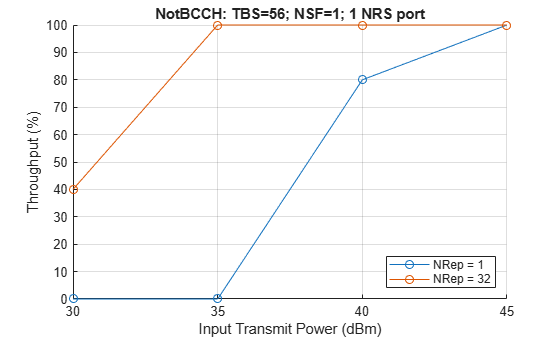This example figure shows the throughput results obtained by simulating 1000 transport blocks (`numTrBlks = 1000`, `txPower = 30:45`) for repetition indices 0 and 5. The simulation setup includes the default higher layer, eNodeB, and NPDSCH configuration with an ETSI Rician channel. To obtain the lines corresponding to the `Tx Doppler Comp.`, you can set `txDopplerCompensator` to `true` and `rxDopplerCompensator` to `false`. For the lines corresponding to the `Rx Doppler Comp.`, you can set `txDopplerCompensator` to `false` and `rxDopplerCompensator` to `true`.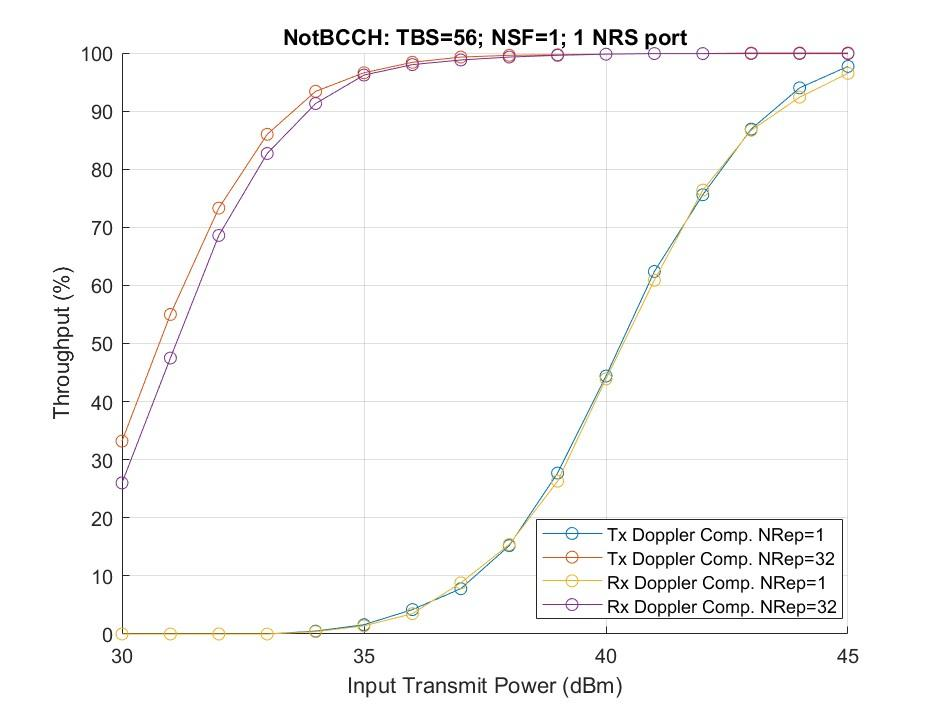### Further Exploration

This example shows the throughput simulation for NB-IoT NPDSCH in an NTN channel. In addition to the default behavior, you can run the example for these cases.

• Analyze the throughput at each transmit power value and repetition index for a different satellite orbit by varying the satellite altitude and satellite speed.

• Observe the link performance without any Doppler compensation techniques by setting the `txDopplerCompensator` and `rxDopplerCompensator` variables to `false`.

• Observe the link performance with only Doppler compensation at only the receiver by setting `txDopplerCompensator` to `false` and `rxDopplerCompensator` to `true`.

• Check the throughput performance for different repetitions by changing the `iReps` value.

• Compare the throughput performance in an NTN and terrestrial network by using the `lteFadingChannel` (LTE Toolbox) channel as shown in NB-IoT NPDSCH Block Error Rate Simulation (LTE Toolbox) example.

### Appendix

This example uses this helper function.

### References

1. ETSI TS 101 376-5-5 V1.3.1 (2005-02). GEO-Mobile Radio Interface Specifications (Release 1); Part 5: Radio interface physical layer specifications; Sub-part 5: Radio Transmission and Reception; GMR-1 05.005.

2. ITU-R Recommendation P.681-11 (08/2019). “Propagation data required for the design systems in the land mobile-satellite service.” P Series; Radio-wave propagation.

3. 3GPP TS 36.211, "Physical channels and modulation", 3rd Generation Partnership Project; Technical Specification Group Radio Access Network; Evolved Universal Terrestrial Radio Access (E-UTRA).

4. 3GPP TS 36.213, "Physical layer procedures", 3rd Generation Partnership Project; Technical Specification Group Radio Access Network; Evolved Universal Terrestrial Radio Access (E-UTRA).

5. 3GPP TS 36.321, "Medium Access Control (MAC) protocol specification", 3rd Generation Partnership Project; Technical Specification Group Radio Access Network; Evolved Universal Terrestrial Radio Access (E-UTRA).

6. 3GPP TS 36.101, "User Equipment (UE) radio transmission and reception", 3rd Generation Partnership Project; Technical Specification Group Radio Access Network; Evolved Universal Terrestrial Radio Access (E-UTRA).

7. 3GPP TS 36.104, "Base Station (BS) radio transmission and reception", 3rd Generation Partnership Project; Technical Specification Group Radio Access Network; Evolved Universal Terrestrial Radio Access (E-UTRA).

8. 3GPP TR 36.763, "Study on Narrow-Band Internet of Things (NB-IoT) / enhanced Machine Type Communication (eMTC) support for Non-Terrestrial Networks (NTN) (Release 17), 3rd Generation Partnership Project; Technical Specification Group Radio Access Network.

9. 3GPP TR 38.803, "Study on new radio access technology: Radio Frequency (RF) and co-existence aspects (Release 14)", 3rd Generation Partnership Project; Technical Specification Group Radio Access Network.

10. 3GPP TR 38.811, "Study on New Radio (NR) to support non-terrestrial networks (Release 15)", 3rd Generation Partnership Project; Technical Specification Group Radio Access Network.

11. O. Hammi, S. Carichner, B. Vassilakis, and F.M. Ghannouchi. “Power Amplifiers’ Model Assessment and Memory Effects Intensity Quantification Using Memoryless Post-Compensation Technique.” IEEE Transactions on Microwave Theory and Techniques 56, no. 12 (December 2008): 3170–79.

### Local Functions

This example uses these local functions.

```function chanOut = setupNTNChannel(channel,sampleRate) % Setup NTN channel % Assign temporary variables for carrier frequency and maximum Doppler % shift due to mobile movement fc = double(channel.CarrierFrequency); c = physconst("LightSpeed"); maxDoppler = (double(channel.MobileSpeed)*fc)/c; elevAngle = double(channel.ElevationAngle); h = double(channel.SatelliteAltitude); v = double(channel.SatelliteSpeed); % Calculate the Doppler shift due to satellite movement maxDopplerSat = satcom.internal.dopplerShift(fc,v,elevAngle,h); % Check the maximum Doppler shift and sample rate if ((maxDoppler+maxDopplerSat) >= (sampleRate/10)) error("satcom:setupNTNChannel:MaxDoppler", ... "The maximum Doppler shift (%d Hz) due to mobile and satellite " + ... "movement, must be less than %d Hz which is one-tenth of SampleRate.", ... (maxDoppler + maxDopplerSat),sampleRate/10) end chanOut = struct; chanTypeLower = lower(channel.NTNChannelType); if chanTypeLower == "etsi rician" channelName = "ETSI Rician"; baseChannel = etsiRicianChannel; baseChannel.SampleRate = sampleRate; baseChannel.KFactor = channel.KFactor; baseChannel.MaximumDopplerShift = maxDoppler; elseif chanTypeLower == "itu-r p.681" channelName = "ITU-R P.681"; baseChannel = p681LMSChannel; baseChannel.SampleRate = sampleRate; baseChannel.Environment = channel.Environment; baseChannel.CarrierFrequency = channel.CarrierFrequency; baseChannel.MobileSpeed = channel.MobileSpeed; baseChannel.ElevationAngle = channel.ElevationAngle; baseChannel.AzimuthOrientation = channel.AzimuthOrientation; baseChannel.FadingTechnique = "Sum of sinusoids"; end baseChannel.RandomStream = "mt19937ar with seed"; baseChannel.Seed = channel.Seed; % Set the channel filter chanFilt = comm.ChannelFilter( ... SampleRate=sampleRate,PathDelays=0, ... NormalizeChannelOutputs=false); % Set the output structure chanOut.ChannelName = channelName; chanOut.CarrierFrequency = fc; chanOut.SatelliteSpeed = v; chanOut.SatelliteAltitude = h; chanOut.ElevationAngle = elevAngle; chanOut.BaseChannel = baseChannel; chanOut.SatelliteDopplerShift = maxDopplerSat; chanOut.ChannelFilter = chanFilt; end function [out,sampleTimes] = generateNTNChannel(channel,in) % Generate NTN channel % Get the channel information before channel processing prevInfo = info(channel.BaseChannel); numSamplesStart = prevInfo.NumSamplesProcessed; % Get the path gains of base channel [~,pathGainsBase] = channel.BaseChannel(in); % Get the channel information after channel processing postInfo = info(channel.BaseChannel); numSamplesEnd = postInfo.NumSamplesProcessed; % Get the channel sample times sampleTimes = (numSamplesStart:(numSamplesEnd-1)).'/channel.BaseChannel.SampleRate; % Apply satellite Doppler shift to the base channel path gains pathGains = pathGainsBase.*exp(1i*2*pi*channel.SatelliteDopplerShift*sampleTimes); % Perform channel filtering out = channel.ChannelFilter(in,pathGains); end function out = compensateDopplerShift(enb,inWave,foffset,flag) % Perform Doppler shift correction if flag % Correct frequency offset out = lteFrequencyCorrect(enb,inWave,foffset); else out = inWave; end end function out = estimateDopplerShiftUsingRS(enb,rxWave,refInd, ... refSym,flag) % Estimate the Doppler shift using NPSS if flag % Set the Windowing field to 0, as this information is not known at % the receiver enb.Windowing = 0; ofdmInfo = lteSCFDMAInfo(enb); K = 12; % Number of subcarriers L = 14; % Number of OFDM symbols in slot % Initialize temporary variables rxWave1 = [rxWave; zeros((mod(size(rxWave,1),2)),1)]; % Append zero, if required rxLen = size(rxWave1,1); % Generate reference waveform refGrid = complex(zeros([K L])); refGrid(refInd) = refSym; refWave = lteSCFDMAModulate(enb,refGrid); refWave = [refWave; zeros((rxLen-size(refWave,1)),1)]; % Compute the correlation of received waveform with reference % waveform x_wave = rxWave1.*conj(refWave); % Compute FFT of the resultant waveform x_fft = fftshift(fft(x_wave)); % FFT bin values fftBinValues = (-rxLen/2:(rxLen/2-1))*(ofdmInfo.SamplingRate/rxLen); % Use the FFT bin index corresponding to the maximum FFT value. % The FFT bin value corresponding to this bin index is the integer % frequency offset. [~,binIndex] = max(x_fft); out = fftBinValues(binIndex); else out = 0; end end function varargout = paMemorylessNonlinearity(paModel,varargin) % Apply power amplifier nonlinearity (TR 38.803) % out = paMemorylessNonlinearity(paModel,in,enable) returns the % impaired output. % paMemorylessNonlinearity(paModel) returns the plot with the gain and % phase characteristics of the power amplifier if nargin == 1 in_NoScale = randn(1e6,1)+1j*randn(1e6,1); scaleFactor = 1/sqrt(2); enable = 1; else in_NoScale = varargin{1}; scaleFactor = 1; enable = varargin{2}; end if enable in = scaleFactor*in_NoScale; if isa(paModel,"comm.MemorylessNonlinearity") % paModel is a comm.MemorylessNonlinearity System object out = paModel(in); paModelName = ""; else absIn = abs(in); paModelName = paModel; switch lower(paModel) case "2.1ghz gaas" % 2.1GHz GaAs out = (-0.618347-0.785905i) * in + (2.0831-1.69506i) * in .* absIn.^(2) + ... (-14.7229+16.8335i) * in .* absIn.^(2*2) + (61.6423-76.9171i) * in .* absIn.^(2*3) + ... (-145.139+184.765i) * in .* absIn.^(2*4) + (190.61-239.371i)* in .* absIn.^(2*5) + ... (-130.184+158.957i) * in .* absIn.^(2*6) + (36.0047-42.5192i) * in .* absIn.^(2*7); otherwise % 2.1GHz GaN out = (0.999952-0.00981788i) * in + (-0.0618171+0.118845i) * in .* absIn.^(2) + ... (-1.69917-0.464933i) * in .* absIn.^(2*2) + (3.27962+0.829737i) * in .* absIn.^(2*3) + ... (-1.80821-0.454331i) * in .* absIn.^(2*4); end end else out = in_NoScale; end if nargout > 0 varargout{1} = out; end if nargin == 1 || (nargout == 0) % Gain Plot inpPower = 20*log10(absIn); gain = 20*log10(abs(out))-inpPower; figure subplot(211) plot(inpPower,gain,".") grid on ylim([-Inf 1]) xlim([-30 0]) xlabel("Normalized input power (dB)") ylabel("Gain (dB)") title("Gain Characteristics of PA Model " + paModelName) % Phase Plot phase = angle(out.*conj(in))*180/pi; subplot(212) plot(inpPower,phase,".") grid on xlim([-30 0]) xlabel("Normalized input power (dB)") ylabel("Phase (deg)") title("Phase Characteristics of PA Model " + paModelName) end end function paChar = getDefaultCustomPA() % The operating specifications for the LDMOS-based Doherty amplifier are: % * A frequency of 2110 MHz % * A peak power of 300 W % * A small signal gain of 61 dB % Each row in HAV08_Table specifies Pin (dBm), gain (dB), and phase shift % (degrees) as derived from figure 4 of Hammi, Oualid, et al. "Power % amplifiers' model assessment and memory effects intensity quantification % using memoryless post-compensation technique." IEEE Transactions on % Microwave Theory and Techniques 56.12 (2008): 3170-3179. HAV08_Table = ... [-35,60.53,0.01; -34,60.53,0.01; -33,60.53,0.08; -32,60.54,0.08; -31,60.55,0.1; -30,60.56,0.08; -29,60.57,0.14; -28,60.59,0.19; -27,60.6,0.23; -26,60.64,0.21; -25,60.69,0.28; -24,60.76,0.21; -23,60.85,0.12; -22,60.97,0.08; -21,61.12,-0.13; -20,61.31,-0.44; -19,61.52,-0.94; -18,61.76,-1.59; -17,62.01,-2.73; -16,62.25,-4.31; -15,62.47,-6.85; -14,62.56,-9.82; -13,62.47,-12.29; -12,62.31,-13.82; -11,62.2,-15.03; -10,62.15,-16.27; -9,62,-18.05; -8,61.53,-20.21; -7,60.93,-23.38; -6,60.2,-26.64; -5,59.38,-28.75]; % Convert the second column of the HAV08_Table from gain to Pout for % use by the memoryless nonlinearity System object. paChar = HAV08_Table; paChar(:,2) = paChar(:,1) + paChar(:,2); end```